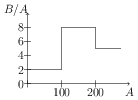# Binding Energy and its Calculation

## Problems from IIT JEE

Problem (IIT JEE 2008):Assume that the nuclear binding energy per nucleon $(B/A)$ versus mass number $(A)$ is as shown in the figure. Use this plot to choose the correct choice(s) given below,

1. Fusion of two nuclei with mass number lying in the range of $1 < A < 50$ will release energy.
2. Fusion of two nuclei with mass number lying in the range of $51 < A < 100$ will release energy.
3. Fission of a nucleus lying in the mass range of $100 < A < 200$ will release energy when broken into two equal fragments.
4. Fission of a nucleus lying in the mass range of $200 < A < 260$ will release energy when broken into two equal fragments.

Solution: If $B/A$ of the products is more than that of the reactants than energy is released in the reaction. Fusion of two nuclei with mass number in $1 < A < 50$ produces a nuclei with mass number in $1 < A < 100$. The $B/A$ of product is equal to that of reactants, hence no energy is released.

Fusion of two nuclei with mass number in $51 < A < 100$ produces a nuclei with mass number in $100 < A < 200$. The $B/A$ of product is more than that of reactants, hence energy is released.

The fission of nucleus with mass number $100 < A < 200$ produces products with mass number lying in $1 < A < 200$. The $B/A$ of products is less than or equal to that of reactants hence no energy is released. The fission of nucleus with mass number $200 < A < 260$ produces products with mass number lying in $100 < A < 200$. The $B/A$ of products is more than that of the reactants, hence energy is released.